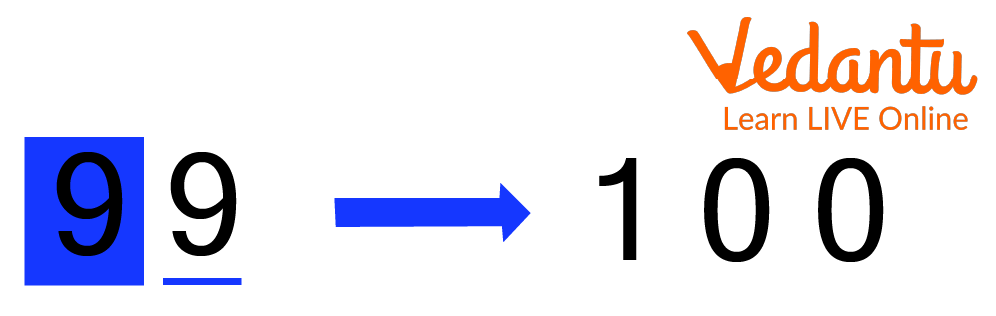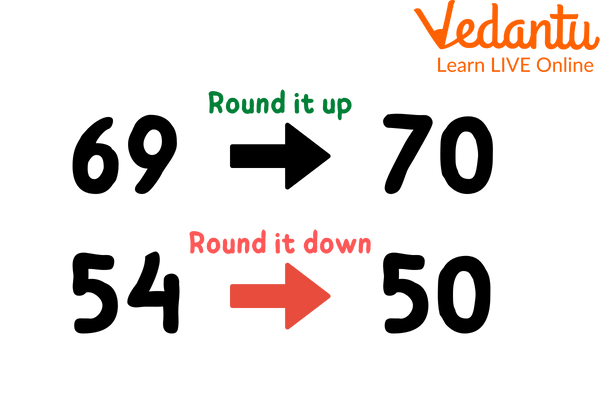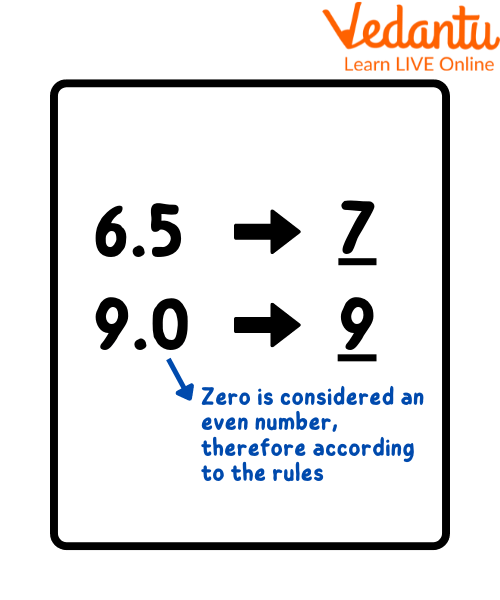Courses
Courses for Kids
Free study material
Free LIVE classes
More

# Rounding Off to the Nearest 100LIVE
Join Vedantu’s FREE Mastercalss

## Introduction to Rounding Off Rules

Have you ever scored an 18.5 out of 20, but your teacher says well done, you have scored a good 19 out of 20, and you are left confused about how a teacher can mistakenly increase your score by 0.5? Actually, it is not a mistake, it is a technique called estimation or rounding off a number to simplify it.Showing Rounding Off of a Number

Rounding off a number means estimating or changing the number to the one it is closest to. Rounding off is a technique used in various subjects like Physics, Chemistry, and Mathematics.

Rounding off implies a number is simplified by changing its worth nearer to the closest number. It may be applied well for any whole number, decimal at different spots of hundreds, tens, tenths, and so on. Rounding off a number is done to maintain the significant figures.

## Significant Figures

A significant figure is the number of positional digits in a number that is reliable and necessary to indicate the necessary quantity or meaning.

The number 23.4 has a total of 3 significant figures. According to the rules, every non-zero digit in a number is always significant. 6.21388 has six significant digits (all the numbers counted as significant give useful information). Thus, 82 has two significant digits, and 82.6 has three significant digits.

## How to Round off: Rounding off Rules

### Rounding Rules for Whole Numbers

To answer the question of how to round off, there are a few simple steps to be always followed. Those are as follows:

• Step 1: To obtain a precise end result, carefully pick the last place value.

• Step 2: Search for the next smallest place, which is towards the right of the number that is being estimated. For instance, if you want to estimate a digit from a tens place, look for a digit at the one's place.

• Step 3: If the digit succeeding the number to be rounded off is under 5, the digit remains unchanged. Any number of digits after that number is converted to zero, and this is called rounding down.

• Step 4: If the digit succeeding the number to be rounded off is greater than 5, the digit is increased by 1 this is called rounding up.

• Step 5: If the digit succeeding the digit to be rounded off is 5 or 5 followed by 0’s, then the figure to be rounded shall be

• incremented by 1 if it is odd, and

• unchanged if even. In this case, 0 is considered an even number.Phenomenon of Rounding Up and Rounding Down a Number

For example, in the above image, 69 is rounding up, and 54 is rounding down by applying the rule discussed in step 5.

## Rounding Rules for Decimal Numbers

• Step 1: Look for the digit to be rounded and look at its preceding number.

• Step 2: If the digits preceding the number to be rounded off are under 5, consider them equal to zero.

• Step 3: If the digits preceding the number to be rounded off are greater than or equivalent to 5, then the number is increased by 1.Rounding Off the Decimals

## Rounding off to the Nearest 100

Let's take the example of the number 4850. To round off this number to its nearest hundreds of places and following are the steps:

• Step 1: First, identify the digit present at the hundreds place: 8

• Step 2: Then, identify the next smallest place in the number: 5

• Step 3: As per the rules, if the smallest place digit is greater than or equivalent to 5, round up the digit. Therefore, add +1 to the digit at the hundreds place. 8+1=9. And the other digits become zero.

Answer: So the final number is 4900.

## Solved Examples

Q 1. Round off 1647 to the nearest hundreds.

Ans: The answer to the question will be 1700

• Step 1: First, identify the digit present at the hundreds place: 6

• Step 2: Then, identify the next smallest place in the number: 4

• Step 3: As per the rules, if the digits preceding the no. to be rounded off are under 5, then consider them equal to zero. Therefore, the digit at the hundreds place remains the same. And the other digits become zero. So the final number is 1600.

Q 2. What is the nearest number after rounding off the following numbers to the nearest hundreds?

1. 1863

2. 4265

3. 430

Ans: Let's round off the following one by one by using the rules of rounding off to a hundred

A. 1863

• Step 1: First, identify the digit present at the hundreds place: 8

• Step 2: Then, identify the next smallest place in the number: 6

• Step 3: As per the rules, if the smallest place digit is greater than or equivalent to 5, round up the digit. Therefore, add +1 to the digit at the hundreds place. 8+1=9. And the other digits become zero. So the final number is 1900.

B. 4265

• Step 1:First, identify the digit present at the hundreds place: 2

• Step 2: Then, identify the next smallest place in the number: 6

• Step 3: As per the rules, if the smallest place digit is greater than or equivalent to 5, then round up the digit. Therefore, add +1 to the digit at the hundreds place. 2+1=3. And the other digits become zero. So the final number is 4300.

C. 430

• Step 1: First, identify the digit present at the hundreds place: 4

• Step 2: Then, identify the next smallest place in the number: 3

• Step 3: As per the rules, if the digit succeeding the number to be rounded off is under 5, the digit remains unchanged. Any number of digits after that number is converted to zero. So the final number is 400.

Q 3. Round the following numbers to the nearest hundred.

1. 3,00,678

2. 13,05,889

Ans:

1.  3,00,678 is the Given Number.

We can determine that the number's digit in the tens position is 7, which is greater than five. Therefore, add a 1 to the hundreds place, and add zeros in the units and tens of the number. Thus, 3,00,700 is the result of rounding 3,00,678 to the nearest hundred.

2. 13,05,889  is the Given Number.

We round to the nearest multiple of the hundred places bigger than the integer when the digit in the tens place is 8, which we see. 13, 05, 889 is closer to 13, 05, 900 than 13, 05, 800.

## Practice Questions

Q 1. Round off 5672.8 to the nearest 100.

Ans: 5700

Q 2. Round of 5681 to the nearest hundreds.

Ans: 5700

Q 3. What is the nearest number after rounding off the following to the nearest 100.

1. 9832

2. 441

Ans: a) 10000 b) 400

## Summary

Keeping a number's value close to its original value while simplifying it is known as rounding. The outcome of rounding off is less precise but simpler, particularly when doing mathematical procedures. Similar to this, rounding a number to the closest hundred entails placing zeros in the units and tens and increasing or decreasing the remainder by one. In the areas of this website, students can find examples of solved problems, definitions, and round-off guidelines.

Last updated date: 20th Sep 2023
Total views: 115.8k
Views today: 2.15k

## FAQs on Rounding Off to the Nearest 100

1. What is the general rule for rounding off?

Round the number up if 5, 6, 7, 8, or 9 follow the number you are rounding. For instance, 38 rounded up to the nearest ten is 40.

Round the number down if it is followed by the digits 0, 1, 2, 3, or 4. For instance, 33 is 30 when rounded to the closest ten.

2. What is the nearest number meant?

The nearest number means that number that is close to the given number. For example, the nearest number to 11 is 10, the nearest number to 79 is 80, nearest number to 999 is 1000.

3. What is the purpose of rounding numbers?

Adjusting a number's digits so that it produces an approximation of a result is known as rounding. When a number is rounded, the result is an approximation that is a simpler and shorter representation of the original number.

4. What does "rounding off" mean?

By keeping the overall value of a number while moving it closer to the next number, rounding off makes it simpler. It is used both for whole numbers and decimals at the tenth, hundredth, thousandth, and other places.